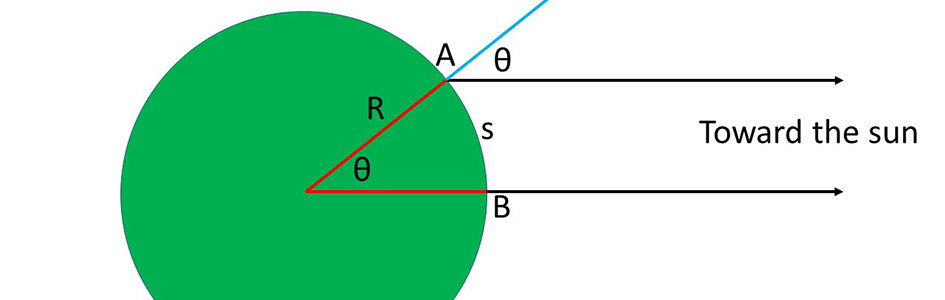# Eratosthenes Reconsidered

on
Share:

In discussions of whether the earth is flat, the work of Eratosthenes often comes up. As I have previously pointed out, many people get Eratosthenes’ famous experiment wrong. It is a common misconception that Eratosthenes proved that the earth is a globe. At the time of Eratosthenes, it was already generally known in the West that the earth is a globe. Eratosthenes built on that knowledge to measure the earth’s circumference and hence its radius.

While there is some uncertainty about the unit of measure that Eratosthenes used, his measurement of the earth’s size is close to the modern accepted value.

How did Eratosthenes do this? The angle that any object in the sky makes with the horizontal is called the altitude. Altitude varies between 0° (on the horizon) and 90° (at the zenith, the point directly overhead). Alternately, we could express this concept as an astronomical body’s zenith angle, the complement of its altitude. If the earth is a globe, then the altitude of the sun at noon on a given date will depend upon how far north or south one is. Therefore, if on the same day the altitude (or zenith distance) of the noonday sun is measured at two locations separated in the north-south direction, one can calculate the earth’s size, provided that the distance between the two locations is known. This is what Eratosthenes did. While there is some uncertainty about the unit of measure that Eratosthenes used, his measurement of the earth’s size is close to the modern accepted value.

Since Eratosthenes already knew the earth was a globe, flat-earthers are correct when they claim Eratosthenes didn’t prove the earth is a globe. Flat-earthers also frequently say that Eratosthenes’ experiment can be explained on a flat earth. This, too, is true. But that explanation has consequences. If the earth is a globe, then the Eratosthenes experiment can be used to measure the earth’s size. But if the earth is flat, the Eratosthenes experiment can be used to measure how high above the flat earth the sun is. Let us compare the results.

## The Original Eratosthenes Experiment

Eratosthenes lived in Alexandria, Egypt, 22 centuries ago. He worked at the famous Great Library that was located there. Alexandria was the logical choice for housing the Great Library because it was the center of Greek culture and learning at the time. Eratosthenes once traveled to Syene in southern Egypt, which was the name of the modern city of Aswan at the time. Eratosthenes noticed that the sun was directly overhead at noon on the June solstice. This is because Syene/Aswan is on the tropic of Cancer, the northernmost edge of the tropics. The sun is directly overhead at local noon on the June solstice for all locations on the tropic of Cancer. Since Alexandria is some 500 miles outside of the tropics, the sun is never overhead there. Eratosthenes understood that this observation provided an opportunity to measure the earth’s size. Upon returning to Alexandria, Eratosthenes constructed a gnomon, a vertical pole of known height over a flat surface. The following year, Eratosthenes measured the length of the gnomon’s shadow at local noon on the June solstice (Figure 1). If the gnomon had height × and the shadow had length y, then the zenith angle, θ, was equal to the arctangent of (y/x). Eratosthenes found the zenith angle of the sun was 1/50 of a circle, or 7.2°.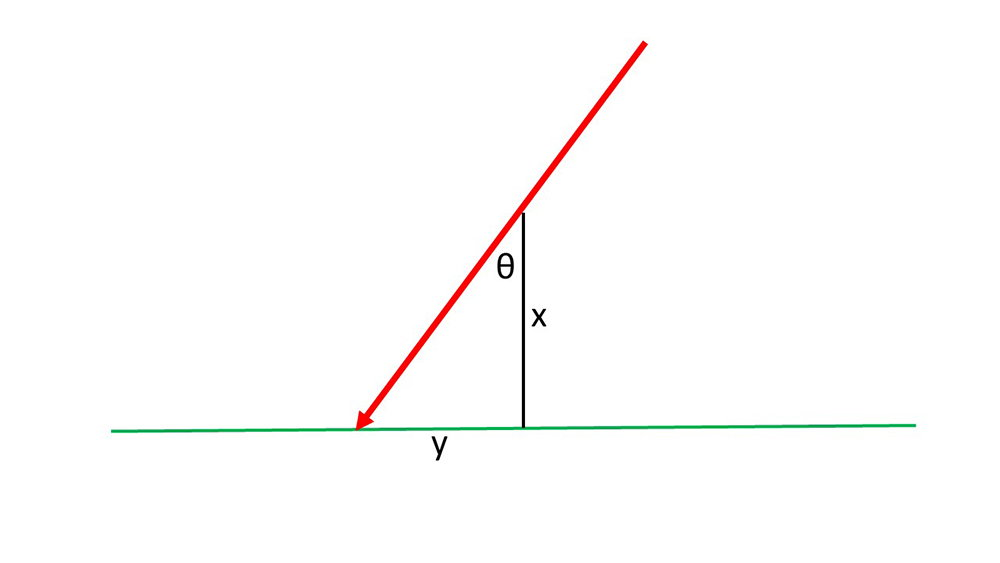Figure 1

Figure 2 shows how Eratosthenes used this information to find the earth’s circumference. Not only did people at the time of Eratosthenes know that the earth is a globe, but they also understood that the sun’s distance was much greater than the earth’s radius. Therefore, Eratosthenes assumed that the sun was far enough away that the direction to the sun at one location on the earth is nearly parallel to the direction to the sun at any other location. Flat-earthers likely will object that the lines are not parallel and so invalidates Eratosthenes’ result. Let us examine that claim. If the sun is 93 million miles away, then the angle between two lines toward the sun from two points on the earth’s surface 500 miles apart is at most 0.0003°. Eratosthenes could not measure an angle that small. The angle he did measure was 24,000 times greater than this. The assumption that the lines are parallel introduced an error of 0.004%. Therefore, this objection is groundless.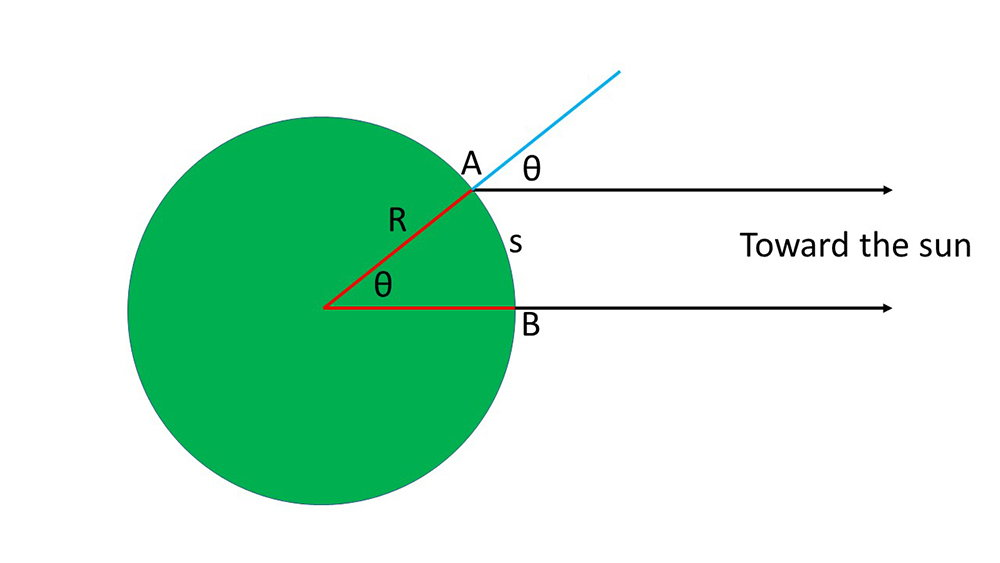Figure 2

From Figure 2, one can see that since the directions to the sun from the two locations are essentially parallel, the zenith angle, θ, must also be the angle between Alexandria (point A) and Syene (point B) measured at the earth’s center. We would say that this angle is the difference in latitude between the two cities. Since θ is 1/50 of a circle, then the arclength, s, between the two cities must be 1/50 of the earth’s circumference. Therefore, the earth’s circumference must be 50 × s. Eratosthenes was the father of geography, so he knew the distance, s, between the two cities. He used a Greek unit of distance called the stadium, but we can use the modern distance between the two cities, 500 miles. Multiplying 50 by 500 miles, we get 25,000 miles for the earth’s circumference. This is within 0.5% of the modern accepted value of the earth’s circumference.

## A Reinterpretation of Eratosthenes’ Result

Now let us reinterpret the Eratosthenes experiment assuming that the earth is flat (Figure 3). If the sun is height h above the earth, and if h is not too great compared to the earth’s size, then the sun’s altitude will be different when measured at the same time at different locations. As before, Alexandria is at point A and Syene is at point B, with distance s between them. The sun is directly overhead at point B, and the sun will have zenith angle θ at point A. Let h be the height of the sun above the flat earth. Then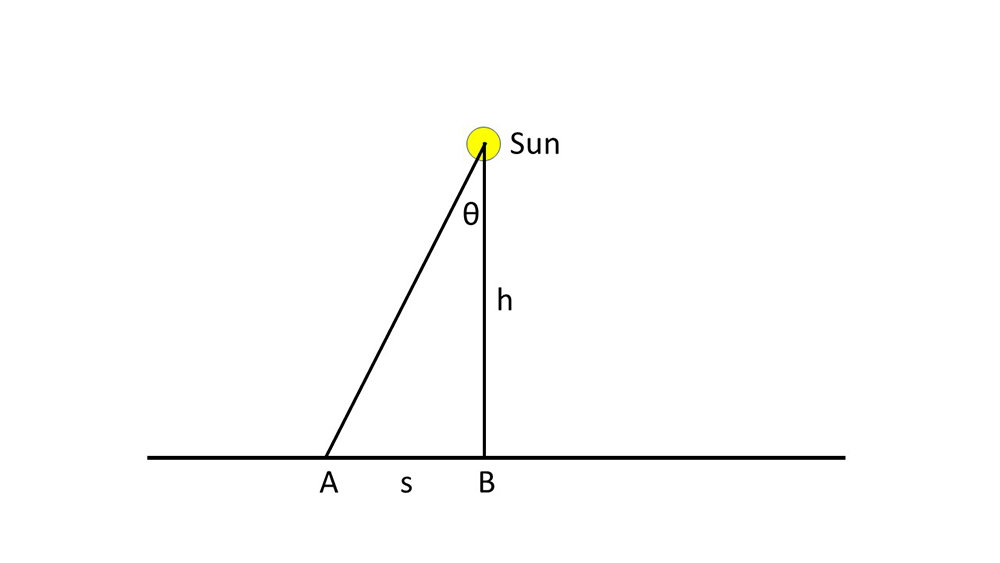Figure 3

Putting in the values, we find that h = 3,958 miles. Expressed as three significant figures, this is 3,960 miles; to two significant figures, it is 4,000 miles. I have found that contemporary flat-earthers avoid any opinion regarding the height of the sun, but when they do opine, a figure in the range of 3,000–4,000 miles is common.1 This figure probably is due to this reinterpretation of the Eratosthenes experiment.

## My Own Eratosthenes-Type Experiment

At local noon on Wednesday, December 9, I conducted an Eratosthenes-type experiment, except that I did this near the December solstice, which was less than two weeks away. Close to either solstice the sun’s declination2 does not change much each day. For instance, at the time of my experiment, the sun’s declination was about -22° 53', which is within 33' (0.55°) of the sun’s minimum declination on the December solstice 12 days later. Since the sun’s day-to-day change in declination is so little around the solstices, doing an Eratosthenes-type experiment near one of the solstices is more forgiving than any other time of the year. Therefore, it is most feasible to conduct this sort of experiment near one of the solstices. In the Northern Hemisphere, the advantage of doing this near the December solstice rather than the June solstice is that with longer shadows, one can measure the zenith distance with greater accuracy.

The sun’s declination at the time of my experiment rounds to 23° very nicely. This means that the sun was directly overhead at noon at locations around 23° S latitude when I did my experiment. Note that this is true whether the earth is flat or a globe. Obviously, I wasn’t able to confirm whether the sun was indeed overhead at 23° S latitude at noon that day, but I have confidence that it was, and I’m sure that most flat-earthers would agree.3 The question is, what is the distance between the 39th N parallel where I live and the 23rd S parallel? Generally, it is understood that there are 69 statute miles between each degree of latitude difference. Therefore, the distance from where I live and the closest place where the sun was directly overhead at noon that day is (39 + 23) × 69 miles = 4,278 miles. If flat-earthers wish to dispute that there are 69 miles for each degree of latitude, then I will be happy to recompute my results for whatever conversion they prefer.

Figure 4 shows my equipment. I fastened a yardstick to the side of wooden box and placed the box on a level surface. This device kept the yardstick perpendicular to the ground. I used a tape measure to record the length of the yardstick’s shadow at local noon (Figure 5). As you can see, the shadow length was 65.5 inches. To ensure that this was the minimum shadow length for the day, I took measurements over more than a half-hour around the time of local noon (accounting for the equation of time as well). The altitude of the sun, θ, is the arctangent of 36/65.5 = 28.8 degrees. Therefore, the zenith angle of the sun at local noon was 61.2 degrees. Using the same reasoning as Eratosthenes used, the earth’s circumference is (360/61.2) × 4,278 miles = 25,162 miles. To two significant figures, this is 25,000 miles, matching the result of Eratosthenes. To three significant figures, it is 25,200 miles. This is well within 1% of Eratosthenes’ value, and within 1.2% of the modern circumference of the earth. Given the likely errors of my measurement, this is good agreement.Figure 4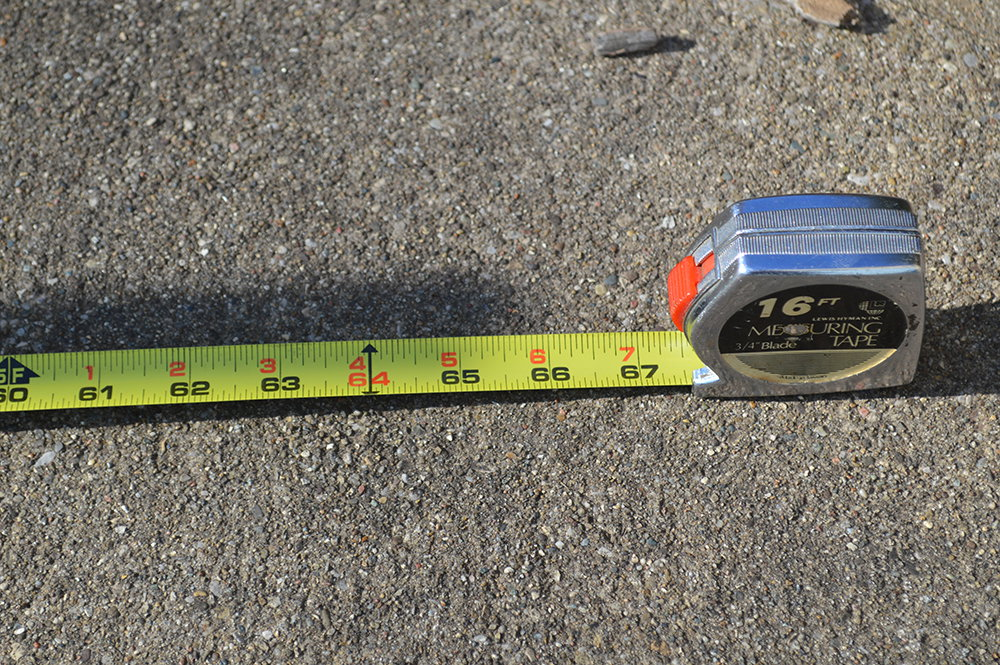Figure 5

What height for the sun results in reinterpreting my result, assuming that the earth is flat? As with the reinterpretation of the original Eratosthenes experiment, the sun’s height will beWith s = 4,278 miles and θ = 28.8°, we find that h = 2,352 miles. Expressed to three significant figures, this is 2,350 miles; to two significant figures, it is 2,400 miles. Note that this is 1,600 miles less than the result of the original Eratosthenes experiment reinterpreted in terms of the earth being flat, a difference of 40%. Contrast that with the very small difference in measuring the earth’s size assuming that the earth is a globe. When large deviations between experimental results occur, it is because there is a fundamental problem. The most likely explanation is that the earth is not flat. Since the experiment gives consistent results assuming that the earth is a globe, this experiment is confirmation of the globe model. Note that a single Eratosthenes-type experiment cannot be used as evidence for the earth being a globe, but multiple tests conducted over various distances is evidence that the earth is a globe.

## History of Flat-Earth Measurements of the Sun’s Height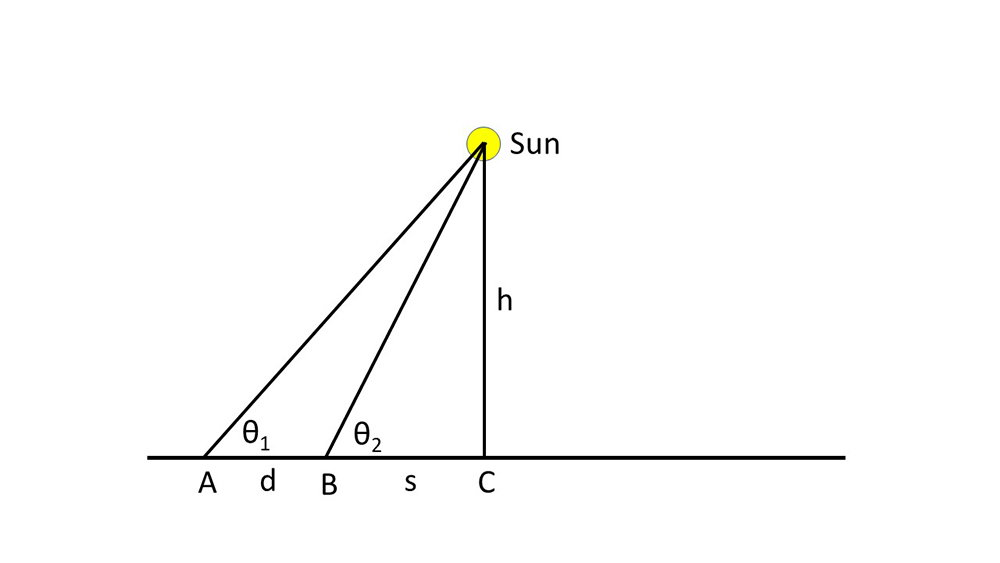Figure 6

Samuel Rowbotham was the founder of the flat-earth movement. In 1865, writing under the pseudonym Parallax, Rowbotham attempted his first measurement of the sun’s height above the earth.4 Rowbotham claimed, “The distance will be thus found to be considerably less than 4,000 miles.” Rowbotham did not provide the calculations for this conclusion, though it is easy enough to do. Figure 6 is an adaptation of Rowbotham’s Figure 26. The sun is directly over the subsolar point C. At the same instant that the sun is over point C, measure the sun’s altitude, θ1, at point A, and the sun’s altitude θ2, at point B. Let d be the distance between points A and B, let s be the distance between points B and C, and let h be the height of the sun above the earth. If we know the angles θ1 and θ2 and the distance d, then h and s are the only unknowns. With basic trigonometry and algebra, one may solve for both unknowns. Since there is a right angle at point C, thenandEliminate h by equating these two equations and then solve for s:Once one solves the third equation for s, the value for s can be substituted into either of the first two equations to find h.

Rowbotham proposed that if such an experiment were conducted at London and 51 miles south at Brighton, the height of the sun “will thus be found to be considerably less than 4,000 miles.” Rowbotham apparently didn’t perform this experiment, for he offered no data. However, he did provide data from such measurements made by the Ordnance Survey (the national mapping agency of Great Britain) at two other locations (London and Ackworth, 151 miles to the north of London) on an unspecified day and time:
θ1 = 53° 02'
θ2 = 55° 13'
d = 151 miles

Putting in the appropriate values into the third equation above, I find s = 1,806 miles. Substituting this back into either of the first two equation yields h = 2,600 miles. Granted, this is “less than 4,000 miles,” but why didn’t Rowbotham say that it was less than 3,000 miles instead? That would be how most people would express it. To characterize 2,600 miles as “less than 4,000 miles” is misleading at best. Could it be that Rowbotham had reinterpreted Eratosthenes’ experiment as I did above and wanted his new computation to match that result? If so, then why didn’t he say so?

Rowbotham gave another example with data again provided by the Ordnance Survey:
θ1 = 38°
θ2 = 45°
d = 800 miles

Note that these measurements lack the precision of the earlier set of data. Using these measurements in the three equations above, I get both h and s equal to 2,858 miles. This is a 9.5% difference from the first example. Rowbotham again concluded that the result is “less than 4,000 miles.”

In his second book, now under his own name, Rowbotham, returned to his hypothetical experiment between London and Brighton conducted at noon on July 13, 1870, and concluded that the sun is 700 miles above the earth5. Rowbotham provided these measurements:
London: θ1 = 61°
Brighton: θ2 = 64°
d = 50 miles

Note that Rowbotham revised the distance from 51 miles to 50 miles, but that is a trivial difference. Unlike in his earlier book, Rowbotham explained how he measured h and s. He solved by construction, making a scale diagram (his Figure 58) and measuring those lengths. Why didn’t Rowbotham use trigonometry and algebra as I did above? Two possibilities immediately come to mind. It could be that Rowbotham lacked these mathematical skills, or it could be that he feared his readers lacked these skills. Using Rowbotham’s measurements, I find s = 366 miles. This is the distance from Brighton to the subsolar point. Since the distance between London and Brighton is 50 miles, the subsolar point is 366 miles + 50 miles = 416 miles from London. Rowbotham said that this distance was 400 miles, an error of only 3.8%. Given that he solved the problem by construction and the possibility of rounding on his part, this is good agreement. For the sun’s height h, I get 751 miles, close to Rowbotham’s 700 miles. This is an error of 6.8%, but, again, given that Rowbotham solved by construction and the inclusion of rounding, this is good agreement. Therefore, we can be confident that Rowbotham correctly determined s and h based upon his data. Rowbotham went on to opine that consideration of atmospheric refraction made it “perfectly safe to affirm that the under edge of the sun is considerably less than 700 statute miles above the earth,” though he did not give justification for this conclusion. Rowbotham went on to claim that based on other, unspecified experiments, the moon is lower than this and that the stars were not more than 1,000 miles above the earth.

Rowbotham properly found the values of s and h, but how reasonable is his result?

Rowbotham properly found the values of s and h, but how reasonable is his result? Rowbotham’s experiment placed the subsolar point 400 miles due south of London. But 400 miles due south of London is a short distance northeast of Bordeaux, France, near the 45th north parallel of latitude. This is more than 20° north of the tropics, so the sun is never directly overhead there. Obviously, Rowbotham’s sun altitude measurements were wrong. For the date Rowbotham specified, I would expect in London the noon sun to have altitude of about 60°, close to Rowbotham’s reported 61°. However, since Brighton is only 50 miles south of London, I would expect the sun’s noon altitude there to be less than a degree greater than the sun’s noon altitude in London, not 64°. Therefore, Rowbotham’s measurement at Brighton clearly was in error. The fact that Rowbotham’s computed distance between London and the subsolar point was ridiculously too short should have been a clue that he was wrong. However, there is no evidence that Rowbotham saw this. Will any current flat-earthers find this to be a problem?

A few other flat-earthers of Rowbotham’s era provided their own estimates of the sun’s height. For instance, in 1893 Alexander Gleason concluded that both the sun and the North Star are 1,725 miles above the earth.6 Gleason did this by using a very confused methodology, but he had no doubt as to it being correct. How is it confused? Gleason stated that there are “60 miles per degree.” This is true of nautical miles, but not statute miles (there are 69 statute miles per degree with one nautical mile equal to 1.1528 statute miles). Most people reading Gleason’s book would think of statute miles, not nautical miles, so this is at best confusing. Unless otherwise specified or in nautical contexts, it is generally understood that the term “mile” refers to statute miles, not nautical miles. I suspect that Gleason didn’t know the difference. Gleason’s argument went downhill from there, making it impossible for me to follow it. For instance, Gleason’s Figure 25 seems to have 2 × 1,725 miles = 3,450 miles between the equator and the North Pole, but since he already said that there were 60 miles per degree and equator is 90 degrees from the North Pole, then that distance ought to be 90 × 60 miles = 5,400 miles. Conversion between nautical and statute miles cannot rectify this.

Gleason went on to say,

Some of the zetetic faith had given the sun’s altitude 2500, 3500, and some even less than I have given it. By what process they have arrived at the various results I do not know, but I know of no other geometrical process than the last herein given.
The discordant results ought to have been an indication that there was something terribly wrong with what Gleason and other flat-earthers of his day were doing.

As I said, Gleason seemed to have had no doubt about the correctness of his method, but he didn’t even begin to address why there was a large range in the estimates of how high the sun is within the flat-earth model. Again, the discordant results ought to have been an indication that there was something terribly wrong with what Gleason and other flat-earthers of his day were doing.

In 1899 Thomas Winship used a method similar to Gleason’s to get a measurement of the sun’s height.7 Winship correctly stated that on the equinoxes the sun is directly overhead at noon on the equator and that the sun has an altitude of 45° at noon at locations at 45° north latitude and 45° south latitude. This is what is expected if the earth is a globe and the sun is much farther away than the earth’s radius. However, as with Eratosthenes’s experiment, one may reinterpret this observation in terms of a flat earth, as Winship did. Winship reasoned that if the earth is flat, then the distance from either 45th parallel to the equator is one leg of a 45-45-90 triangle, with the other leg being the sun’s height. The legs of a 45-45-90 triangle are the same length, so the sun’s height must be the distance between a 45th parallel and the equator. Stating that “60 geographical miles = 1 degree,” Winship concluded that the sun is 45 × 60 geographical miles = 2,700 geographical miles above the earth. “Geographical mile” is an alternate term for nautical mile, so the Winship’s calculation is that the sun is 3,113 statute miles above the earth. We could round this to either 3,110 miles or 3,100 miles.

## Taking Winship’s Method a Step Further

It would have been interesting if Winship would have applied this reasoning to one of the solstices rather than just on the equinoxes (thus making it an Eratosthenes-type experiment as I did above). For instance, at noon on the June solstice, the sun will have an altitude of 68.4° at latitude 45° north. Meanwhile, the sun will be directly overhead at noon at latitude 23.4° north latitude. This is a difference in distance of (45 – 23.4) × 60 nautical miles = 1,296 nautical miles. The sun’s height would be 1,296 nautical miles × tangent (68.4°) = 3,273 nautical miles = 3,773 statute miles. This is 660 statute miles higher than Winship’s claim. If one considers the same experiment at noon on the December solstice at 45° south latitude, the situation will be the same with the same computation of the sun’s height.

But what if we did the same experiment at 45° with the opposite solstice? That is, what if we performed this experiment at noon on the December solstice at 45° north latitude or on the June solstice at 45° south latitude? The sun would have an altitude of 21.6°, and the difference in distance would be (45 + 23.4) × 60 nautical miles = 4,104 nautical miles. This results in the sun’s height being 4,104 nautical miles × tangent (21.6°) = 1,625 nautical miles = 1,873 statute miles, 1,240 miles less than Winship’s result.

Granted, I didn’t measure the altitude of the sun at 45° latitude on either solstice, but keep in mind that Winship didn’t actually measure any altitudes of the sun either. Rather, I computed the sun’s altitude for time, date, and latitude using the globe model. This amounted to a prediction of the globe model that anyone can check, and I encourage others, and especially flat-earthers, to check my predictions by making these observations.

In the meantime, let us use Winship’s height of the sun to predict what the sun’s altitude ought to be at noon on the December solstice at 45° north latitude. The distance between 45° north latitude and the tropic of Capricorn, where the sun is overhead at noon on the December solstice is (45 + 23.4) × 60 nautical miles = 4,104 nautical miles. If the sun’s height is 2,700 nautical miles, then the sun’s altitude will be arctangent (2,700/4,104) = 33.4°. Recall that I measured the noontime altitude of the sun at 39° north latitude near the December solstice to be 28.8°. How is that at noon on a day near the December solstice the sun can be 4.5° higher in the sky at a location 6° farther north from where I live? The obvious answer is that it can’t. Therefore, there is something terribly wrong with Winship’s model. The earth is not flat.

## Conclusion

The inconsistent results of the sun’s height indicate that the earth is not flat.

I have demonstrated that interpreting various measurements of the sun’s altitude assuming the earth is flat leads to widely varying heights of the sun above the earth. Flat-earthers themselves have gotten a wide range of measurements of the sun’s height. The inconsistent results of the sun’s height indicate that the earth is not flat. On the other hand, the consistent results of measurements of the earth’s radius assuming that the earth is spherical confirms the hypothesis that the earth is a globe. Hence the globe earth is established by science, while science disproves the notion that the earth is flat.

Unlike flat-earthers from more than a century ago, contemporary flat-earthers avoid taking a position on the sun’s height above the earth because many of them realize that if they do, their height of the sun can be used to make testable predictions. Flat-earthers tout experiments that produce results that they think confirm that the earth is flat, thus in their minds proving that their model is true. But they are extremely selective, concentrating on a few experiments that they like while simultaneously ignoring many other results that contradict what they have chosen to believe. This is not how science works.

## Footnotes

1. However, some current flat-earthers believe that the sun is no higher than the clouds, a matter of just a few miles above the earth.
2. Declination is a measure how far north or south of the celestial equator a celestial object is.
3. Keep in mind that Eratosthenes didn’t observe the sun to be directly overhead at noon on the same day he was conducting his experiment in Alexandria either. Apparently it was common knowledge in Syene that the sun always was at the zenith at noon on the June solstice.
4. Parallax, Zetetic Astronomy: Earth Not a Globe (London: Simpkin, Marshall, and Company, 1865), 72-74.
5. Samuel Birley Rowbotham, Zetetic Astronomy: Earth Not a Globe (London: Forgotten books, 1881, reprinted 2007), 79–81.
6. Alexander Gleason, Is the Earth a Globe? (Winder, Georgia: Sacred Word Publishing, 1893, reprint), 64–66 (317–319 in the original).
7. Thomas Winship, 1899. Zetetic Astronomy: That the World is Not a Rotating Revolving Globe (Winder, Georgia: Sacred Word Publishing, 1899), 197–201.

### Danny Faulkner’s Blog Blog Updates

Email me with new blog posts by Danny Faulkner’s Blog: